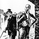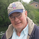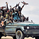TradingView6342瀏覽
RSI Donchian Channels

Built by Ricardo and JR

Here is a great indicator to use for strong trends. Donchian Channels react immediately to changes in the highest high and lowest low. For strong trends you want to trade when RSI is set along the upper or lower DC-RSI envelope . When the RSI releases from the DC-RSI envelope , you can take the trade off.

study(title="[RS][JR]RSI Donchian Channels", shorttitle="[RS][JR]RSI DC", overlay=false)
src = input(defval=close, type=source, title="RSI Source:")
rsi_length = input(defval=14, minval=1, title="RSI Period Length:")
donchian_length = input(20, minval=1, title="Donchian Lookback Period Length:")

rsi1 = rsi(src, rsi_length)

lower = lowest(rsi1, donchian_length)
upper = highest(rsi1, donchian_length)
basis = avg(upper, lower)

plot(rsi1, color=aqua)
l = plot(lower, color=blue)
u = plot(upper, color=blue)
plot(basis, color=orange)
fill(u, l, color=blue)

hline(0)
hline(50)
hline(100)

## 評論The chart published uses close default value candle. You can also use HLC3 for a more current trend trade.Would it be possible to program this to look like a dashboard (?)

For example:
When RSI is set along the upper DC-RSI envelope - it shows green.
When RSI is set along the lower - it shows red.
When its not touching either side it's greyGreat job! Thanks for posting. I like minimal panel space for non-overlays so turned off the 0 and 100 hlines to get good readability (uses only the vertical scale needed so no margins above and below actual plots). Thanks again and keep smiling!Is there a way to set up alerts on this? Would like to automate a test strategySNOW_CITY
@SNOW_CITY, The alerts in tview let you pick many interactions on an indicator. On this one I would give a title to the 4 plots "upper", "lower", "basis" and "rsi" so you can pick the actions wanted in the alert you are setting up. With titles it (instead of Plot Plot Plot Plot and guessing which is which) an easy one would be rsi crossing below basis - when you are awaiting a breakdown swing for example.